## The length of a rectangle is 13 centimeters less than three times its width. Its area is 56 square centimeters. Find the dimensions of the r

Question

The length of a rectangle is 13 centimeters less than three times its width. Its area is 56 square centimeters. Find the dimensions of the rectangle. Use the​ formula, area=length*width.

in progress 0
6 months 2021-07-19T08:45:57+00:00 2 Answers 4 views 0

The dimensions of the rectangle are 8 by 7 centimeters.

Step-by-step explanation:

The length of a rectangle is 13 centimeters less than three times its width. In other words: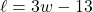Given that the area of the rectangle is 56 square centimeters, we want to determine its dimensions.

Recall that the area of a rectangle is given by: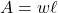Substitute in known values and equations: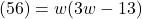Solve for w. Distribute: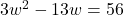Isolate the equation: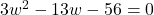Factor. We want to find two numbers that multiply to 3(-56) = -168 and that add to -13.

-21 and 8 suffice. Hence: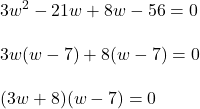Zero Product Property: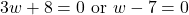Solve for each case: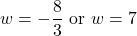Since the width cannot be negative, we can ignore the first solution.

Therefore, the width of the rectangle is seven centimeters.

Thus, the length will be: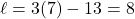Thus, the dimensions of the rectangle are 8 by 7 centimeters.

Area = length  x width

Area = (3width – 13) x width

Area = 3 width^2 -13width

56 = 3width^2 -13 width

Width = 7

Length = (3 *7) -13 = 8

Step-by-step explanation: